# JavaScript中二叉树（二叉堆）的介绍（代码示例）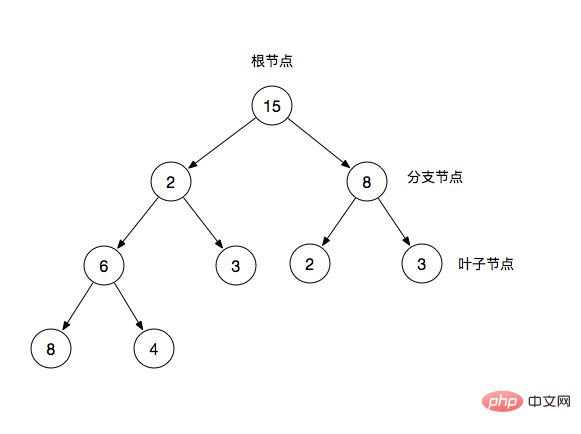• 根节点：二叉树最顶层的节点

• 分支节点：除了根节点以外且拥有叶子节点

• 叶子节点：除了自身，没有其他子节点

1. 在二叉树的第i层上，至多有2^i-1个节点

• i=1时，只有一个根节点，2^(i-1) = 2^0 = 1

2. 深度为k的二叉树至多有2^k-1个节点

• i=2时，2^k-1 = 2^2 - 1 = 3个节点

3. 对任何一棵二叉树T，如果总结点数为n0，度为2(子树数目为2)的节点数为n2,则n0=n2+1

### 树和二叉树的三个主要差别

• 树的节点个数至少为1，而二叉树的节点个数可以为0

• 树中节点的最大度数(节点数量)没有限制,而二叉树的节点的最大度数为2

• 树的节点没有左右之分，而二叉树的节点有左右之分

### 二叉树分类

• 满二叉树：一棵深度为k且有2^k - 1个节点的二叉树称为满二叉树

• 完全二叉树：完全二叉树是指最后一层左边是满的，右边可能满也可能不满，然后其余层都是满的二叉树称为完全二叉树(满二叉树也是一种完全二叉树)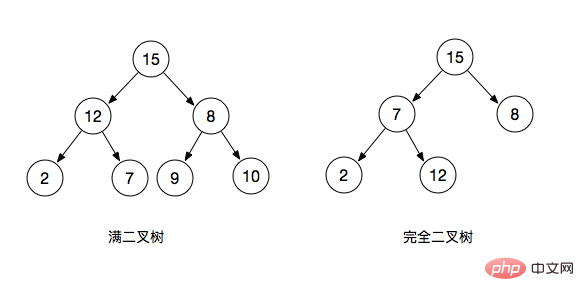### 二叉树的数组表示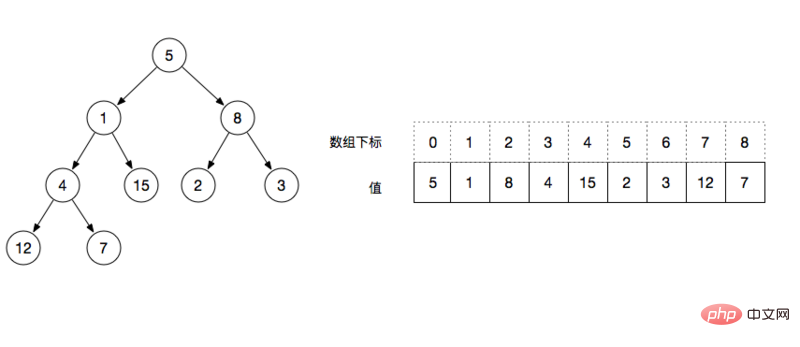• left = index * 2 + 1，例如：根节点的下标为0，则左节点的值为下标array[0＊2+1]=1

• right = index * 2 + 2，例如：根节点的下标为0，则右节点的值为下标array[0＊2+2]=2

• 序数 >= floor(N/2)都是叶子节点，例如：floor(9/2) = 4，则从下标4开始的值都为叶子节点

### 二叉堆

• 二叉堆的父节点的键值总是大于或等于(小于或等于)任何一个子节点的键值

• 当父节点的键值大于或等于(小于或等于)它的每一个子节点的键值时，称为最大堆（最小堆）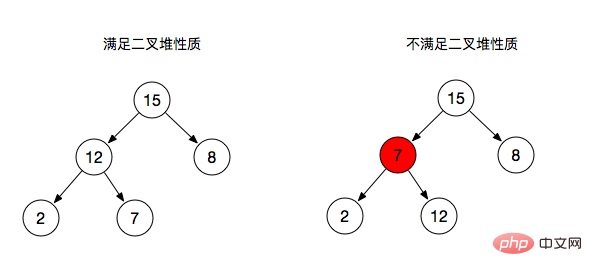• 左图：父节点总是大于或等于其子节点，所以满足了二叉堆的性质，

• 右图：分支节点7作为2和12的父节点并没有满足其性质(大于或等于子节点)。

### 二叉堆的主要操作

• insert：插入节点

• delete：删除节点

• max-hepify：调整分支节点堆性质

• rebuildHeap：重新构建整个二叉堆

• sort：排序

### 初始化一个二叉堆

• 初始化一个数组结构

• 保存数组长度

```    class Heap{
constructor(arr){
this.data = [...arr];
this.size = this.data.length;
}
}```

### max-heapify最大堆操作

max-heapify是把每一个不满足最大堆性质的分支节点进行调整的一个操作。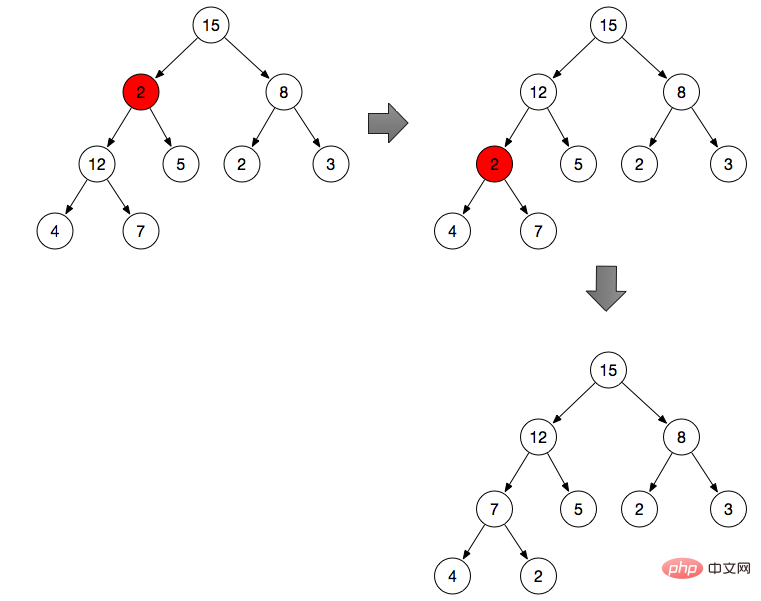1. 调整分支节点2（分支节点2不满足最大堆的性质）

• 默认该分支节点为最大值

2. 将2与左右分支比较，从2，12，5中找出最大值，然后和2交换位置

• 根据上面所将的二叉堆性质，分别得到分支节点2的左节点和右节点

• 比较三个节点，得到最大值的下标max

• 如果该节点本身就是最大值，则停止操作

• 将max节点与父节点进行交换

3. 重复step2的操作，从2，4，7中找出最大值与2做交换

• 递归

```    maxHeapify(i) {
let max = i;

if(i >= this.size){
return;
}
// 当前序号的左节点
const l = i * 2 + 1;
// 当前需要的右节点
const r = i * 2 + 2;

// 求当前节点与其左右节点三者中的最大值
if(l < this.size && this.data[l] > this.data[max]){
max = l;
}
if(r < this.size && this.data[r] > this.data[max]){
max = r;
}

// 最终max节点是其本身,则已经满足最大堆性质，停止操作
if(max === i) {
return;
}

// 父节点与最大值节点做交换
const t = this.data[i];
this.data[i] = this.data[max];
this.data[max] = t;

// 递归向下继续执行
return this.maxHeapify(max);
}```

### 重构堆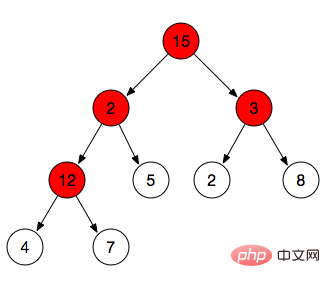• 找到所有分支节点：上面堆的性质提到过叶子节点的序号>=Math.floor(n/2)，因此小于Math.floor(n/2)序号的都是我们需要调整的节点。

• 例如途中所示数组为[15,2,3,12,5,2,8,4,7] => Math.floor(9/2)=4 => index小于4的分别是15，2，3，12(需要调整的节点)，而5，2，8，4，7为叶子节点。

• 将找到的节点都进行maxHeapify操作

```    rebuildHeap(){
// 叶子节点
const L = Math.floor(this.size / 2);
for(let i = L - 1; i>=0; i--){
this,maxHeapify(i);
}
}```

### 最大堆排序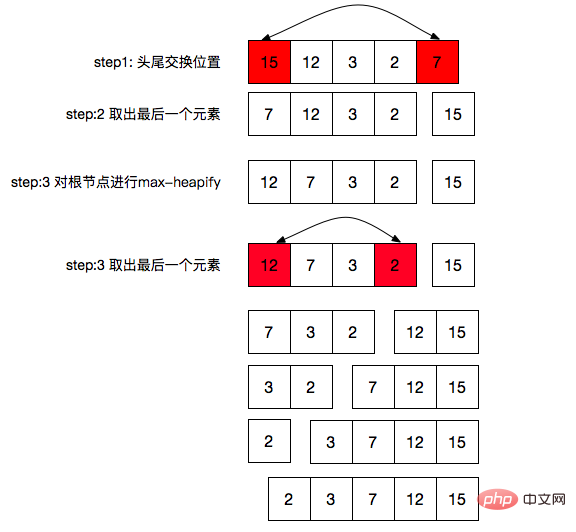• 交换首尾位置

• 将最后个元素从堆中拿出，相当于堆的size-1

• 然后在堆根节点进行一次max-heapify操作

• 重复以上三个步骤，知道size=0 (这个边界条件我们在max-heapify函数里已经做了)

```    sort() {
for(let i = this.size - 1; i > 0; i--){
swap(this.data, 0, i);
this.size--;
this.maxHeapify(0);
}
}```

### 插入和删除

• 往末尾插入

• 堆长度+1

• 判断插入后是否还是一个最大堆

• 不是则进行重构堆

```  insert(key) {
this.data[this.size] = key;
this.size++
if (this.isHeap()) {
return;
}
this.rebuildHeap();
}```
• 删除数组中的某个元素

• 堆长度-1

• 判断是否是一个堆

• 不是则重构堆

```  delete(index) {
if (index >= this.size) {
return;
}
this.data.splice(index, 1);
this.size--;
if (this.isHeap()) {
return;
}
this.rebuildHeap();
}```

### 完整代码

```/**
* 最大堆
*/

function left(i) {
return i * 2 + 1;
}

function right(i) {
return i * 2 + 2;
}

function swap(A, i, j) {
const t = A[i];
A[i] = A[j];
A[j] = t;
}

class Heap {
constructor(arr) {
this.data = [...arr];
this.size = this.data.length;
}

/**
* 重构堆
*/
rebuildHeap() {
const L = Math.floor(this.size / 2);
for (let i = L - 1; i >= 0; i--) {
this.maxHeapify(i);
}
}

isHeap() {
const L = Math.floor(this.size / 2);
for (let i = L - 1; i >= 0; i++) {
const l = this.data[left(i)] || Number.MIN_SAFE_INTEGER;
const r = this.data[right(i)] || Number.MIN_SAFE_INTEGER;

const max = Math.max(this.data[i], l, r);

if (max !== this.data[i]) {
return false;
}
return true;
}
}

sort() {
for (let i = this.size - 1; i > 0; i--) {
swap(this.data, 0, i);
this.size--;
this.maxHeapify(0);
}
}

insert(key) {
this.data[this.size++] = key;
if (this.isHeap()) {
return;
}
this.rebuildHeap();
}

delete(index) {
if (index >= this.size) {
return;
}
this.data.splice(index, 1);
this.size--;
if (this.isHeap()) {
return;
}
this.rebuildHeap();
}

/**
* 堆的其他地方都满足性质
* 唯独跟节点，重构堆性质
* @param {*} i
*/
maxHeapify(i) {
let max = i;

if (i >= this.size) {
return;
}

// 求左右节点中较大的序号
const l = left(i);
const r = right(i);
if (l < this.size && this.data[l] > this.data[max]) {
max = l;
}

if (r < this.size && this.data[r] > this.data[max]) {
max = r;
}

// 如果当前节点最大，已经是最大堆
if (max === i) {
return;
}

swap(this.data, i, max);

// 递归向下继续执行
return this.maxHeapify(max);
}
}

module.exports = Heap;```